Precalculus : Plot Points

Example Questions

Example Question #1 : Plot Points

The point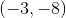is in which quadrant?

IV

II

III

Lies on an axis

I

III

Explanation:

In order to determine in which quadrant the point lies, we must remember the order of the quadrants. The first quadrant is that where x and y are both positive, to the upper right of the origin. To move sequentially to the final quadrant, we go counterclockwise from the first quadrant, which means the second is where x is negative and y is positive, the third is where x and y are both negative, and the fourth is where x is positive and y is negative. We can see from our point (-3,-8) that x and y are both negative, which means the point lies in the third quadrant.

Example Question #2 : Plot Points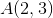andare located on the circle, with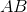forming its diameter. What is the area of the circle.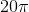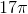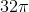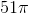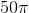Explanation:

Use the distance formula to find the length of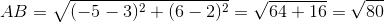.

Since the length ofis that of the diameter, the radius of the circle is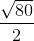.

Thus, the area of the circle is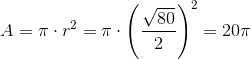.

Example Question #3 : Plot Points

Which of the following coordinates does NOT fit on the graph of the corresponding function?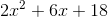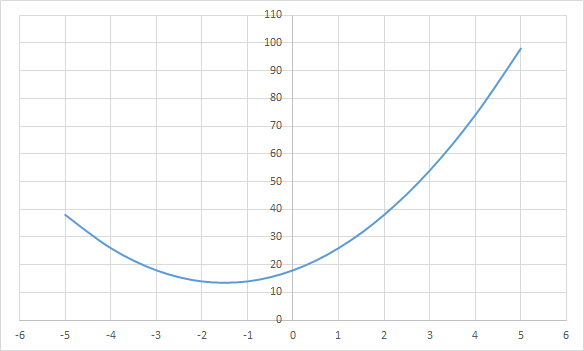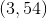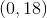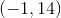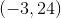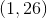Explanation:

When looking at the graph, it is clear that when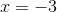,has a value less than. If we were to plug in the value of, our equation would come out as such: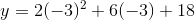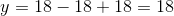Therefore, at, we get a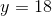, providing the coordinate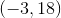.

Example Question #2 : Plot Points

Which of the following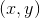coordinates does NOT correspond with the given function and graph?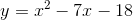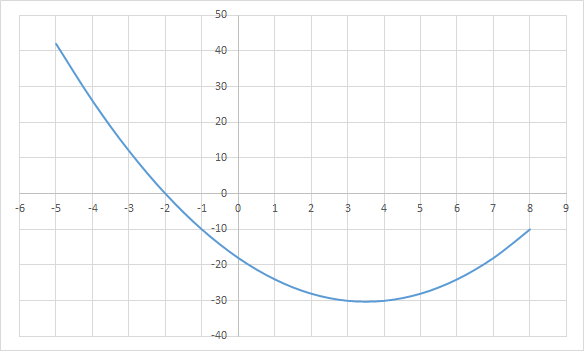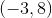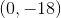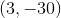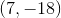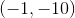Explanation:

When looking at the graph, it is clear that when,has a value greater than. When we plug in bothandvalues into the function, it is clear that these values do not work for the function: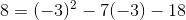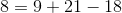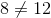Example Question #1 : Cartesian Coordinate System

Which of the followingcoordinates does NOT correspond with the given function and graph?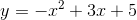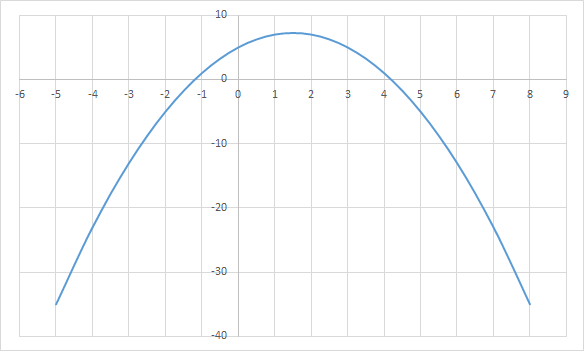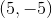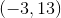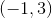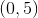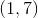Explanation:

If we are to pluginto our function, the values would not work and both sides of the equation would not be equal: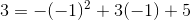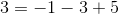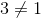Therefore, we know that these coordinates do not lie on the graph of the function.

Example Question #6 : Plot Points

Which of the followingcoordinates does NOT correspond with the given function and graph?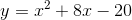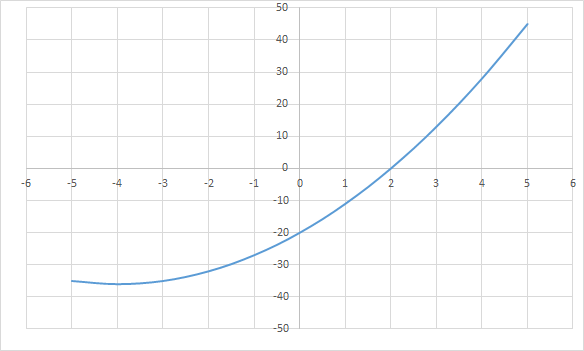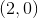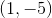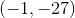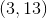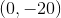Explanation:

If we were to plug in the coordinate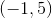into the function, we will find that it does not equate properly: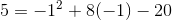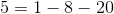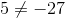Since these values do not equate properly when plugged into the function, we now know thatdoes not fit on the provided graph.

All Precalculus Resources